# How to Sort CSV by multiple columns in Python ?

• Last Updated : 21 Apr, 2021

In this article, we are going to discuss how to sort a CSV file by multiple columns. First, we will convert the CSV file into a data frame then we will sort the data frame by using the sort_values() method.

Syntax: DataFrame.sort_values(by, axis=0, ascending=True, inplace=False, kind=’quicksort’, na_position=’last’)

Attention geek! Strengthen your foundations with the Python Programming Foundation Course and learn the basics.

To begin with, your interview preparations Enhance your Data Structures concepts with the Python DS Course. And to begin with your Machine Learning Journey, join the Machine Learning - Basic Level Course

Return Type: Returns a sorted Data Frame with same dimensions as of the function caller Data Frame.

After converting the CSV file into a data frame, we need to add two or more column names of the CSV file as by parameter in sort_values() method with axis assigned to 0 like below:

sort_values(‘column1’, ‘column2’…’columnn’, axis=0)

CSV file in use: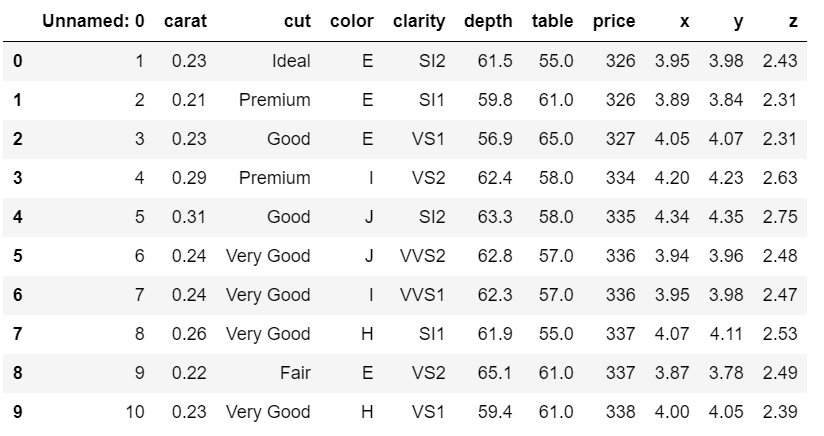Below are various examples that depict how to sort a CSV file by multiple columns:

Example 1:

In the below program, we first convert the CSV file into a dataframe, then we sort the dataframe by a single column in ascending order.

## Python3

 `# importing pandas package ` `import` `pandas as pd ` ` `  `# making data frame from csv file ` `data ``=` `pd.read_csv(``"diamonds.csv"``) ` ` `  `# sorting data frame by a column ` `data.sort_values(``"carat"``, axis``=``0``, ascending``=``True``, ` `                 ``inplace``=``True``, na_position``=``'first'``) ` ` `  `# display ` `data.head(``10``) `

Output: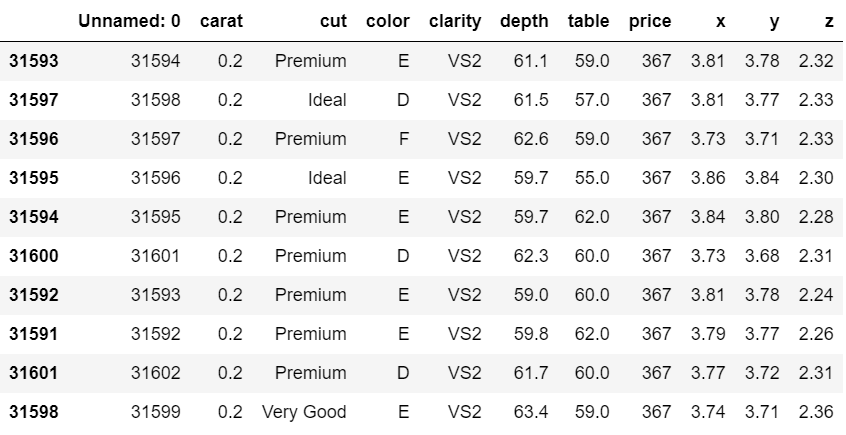Example 2:

Here, after converting into a data frame, the CSV file is sorted by multiple columns, the depth column is sorted first in ascending order, then the table column is sorted in ascending order for every depth.

## Python3

 `# importing pandas package ` `import` `pandas as pd ` ` `  `# making data frame from csv file ` `data ``=` `pd.read_csv(``"diamonds.csv"``) ` ` `  `# sorting data frame by multiple columns ` `data.sort_values([``"depth"``, ``"table"``], axis``=``0``, ` `                 ``ascending``=``True``, inplace``=``True``) ` ` `  `# display ` `data.head(``10``) `

Output: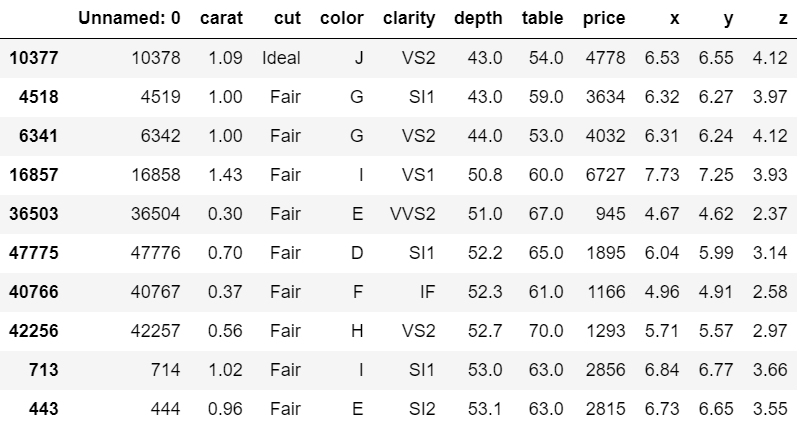Example 3:

In the below example, the CSV file is sorted in descending order by the depth and then in ascending order by the table for every depth.

## Python3

 `# importing pandas package ` `import` `pandas as pd ` ` `  `# making data frame from csv file ` `data ``=` `pd.read_csv(``"diamonds.csv"``) ` ` `  `# sorting data frame by multiple columns ` `data.sort_values([``"depth"``, ``"table"``], axis``=``0``, ` `                 ``ascending``=``[``False``, ``True``], inplace``=``True``) ` ` `  `# display ` `data.head(``10``) `

Output: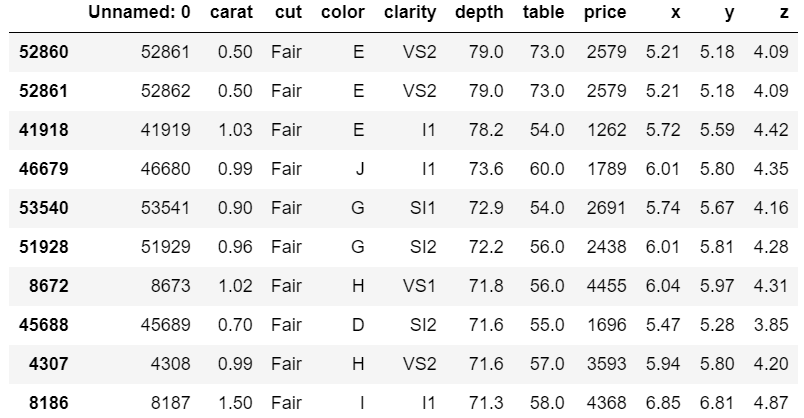Example 4:

Here is another example where the CSV file is sorted by multiple columns.

## Python3

 `# importing pandas package ` `import` `pandas as pd ` ` `  `# making data frame from csv file ` `data ``=` `pd.read_csv(``"diamonds.csv"``) ` ` `  `# sorting data frame by multiple columns ` `data.sort_values([``"depth"``, ``"table"``, ``"carat"``], axis``=``0``, ` `                 ``ascending``=``[``False``, ``True``, ``False``], inplace``=``True``) ` ` `  `# display ` `data.head(``10``) `

Output: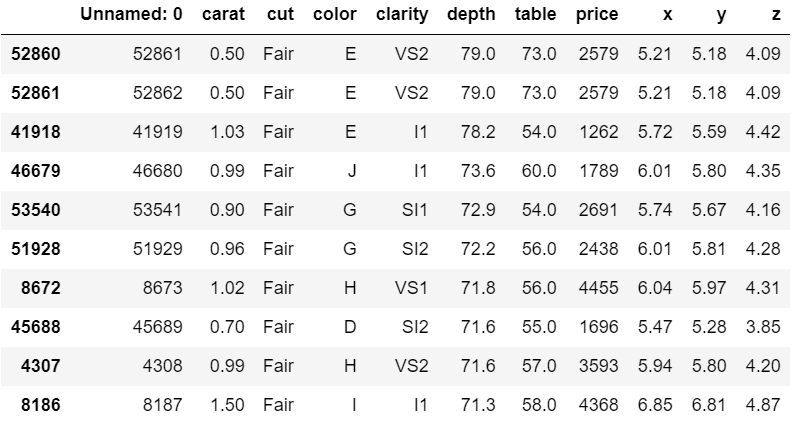My Personal Notes arrow_drop_up
Recommended Articles
Page :